StatLect

Multinoulli distribution

The Multinoulli distribution (sometimes also called categorical distribution) is a generalization of the Bernoulli distribution. If you perform an experiment that can have only two outcomes (either success or failure), then a random variable that takes value 1 in case of success and value 0 in case of failure is a Bernoulli random variable. If you perform an experiment that can haveoutcomes and you denote bya random variable that takes value 1 if you obtain the-th outcome and 0 otherwise, then the random vectordefined asis a Multinoulli random vector. In other words, when the-th outcome is obtained, the-th entry of the Multinoulli random vectortakes value, while all other entries take value.

In what follows the probabilities of thepossible outcomes will be denoted by.Definition

The distribution is characterized as follows.

Definition Letbe adiscrete random vector. Let the support ofbe the set ofvectors having one entry equal toand all other entries equal to: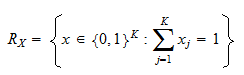Let, ...,bestrictly positive numbers such thatWe say thathas a Multinoulli distribution with probabilities, ...,if its joint probability mass function is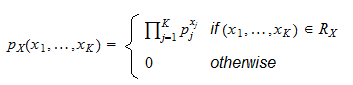If you are puzzled by the above definition of the joint pmf, note that whenandbecause the-th outcome has been obtained, then all other entries are equal toand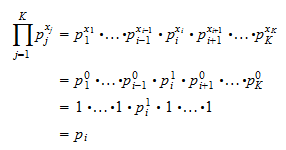Expected value

The expected value ofiswhere thevectoris defined as follows:Proof

The-th entry of, denoted by, is an indicator function of the event "the-th outcome has happened". Therefore, its expected value is equal to the probability of the event it indicates:Covariance matrix

The covariance matrix ofiswhereis amatrix whose generic entry is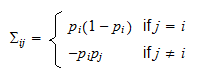Proof

We need to use the formula (see the lecture entitled Covariance matrix):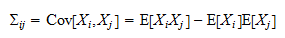If, thenwhere we have used the fact thatbecausecan take only valuesand. If, thenwhere we have used the fact that, becauseandcannot be both equal toat the same time.

Joint moment generating function

The joint moment generating function ofis defined for any:Proof

If the-th outcome is obtained, thenforandfor. As a consequence,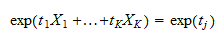and the joint moment generating function is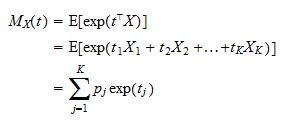Joint characteristic function

The joint characteristic function ofisProof

If the-th outcome is obtained, thenforandfor. As a consequence,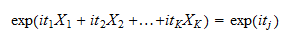and the joint characteristic function is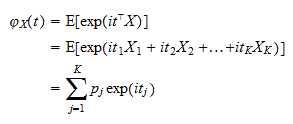More details

The following sections contain more details about the Multinoulli distribution.

Relation between the Multinoulli and the multinomial distribution

A sum of independent Multinoulli random variables is a multinomial random variable. This is discussed and proved in the lecture entitled Multinomial distribution.

The book

Most of the learning materials found on this website are now available in a traditional textbook format.

Glossary entries
Share